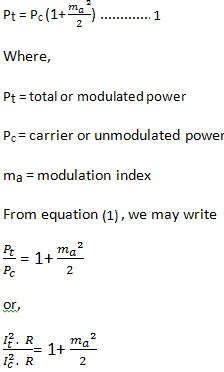# Derive the Current Relations for Single-tone AM (Amplitude Modulated) Wave

## Current Calculation For Single-Tone AM

### Definition

In AM it is generally more convenient to measure the AM transmitter current than the power.

In this case, the modulation index may be calculated from the values of unmodulated and modulated currents in the AM transmitter.

### Mathematical Expression

Let Ic be the r.m.s. value of the carrier or unmodulated current and It be the r.m.s. value of the total or modulated current of an AM transmitter.

Let R be the antenna resistance through which these currents flow.

Now, we know that for a single-tone modulation the power relation is expressed as :